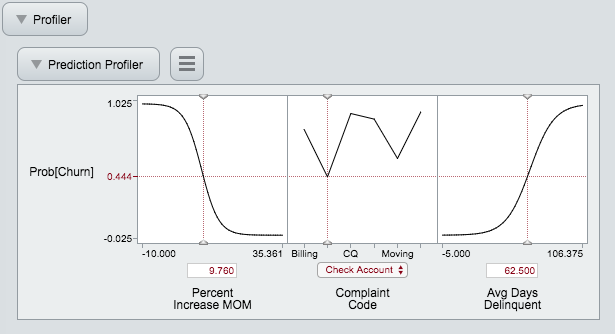It’s World Statistics Day! To honor the theme of the day, the JMP User Community is having conversations about the importance of trust in statistics and data. And we want to hear from you! Tell us the steps you take to ensure that your data is trustworthy.
Choose Language Hide Translation Bar
Interactive HTML Nominal Logistic Regression Profiler Figure

The Nominal Logistic Regression Report has a  Profiler for showing the probability of a binomial outcome as a function of your model effects. This is a great figure, but when its exported using the "Interactive HTML" option, the figure is static. These are some quick notes on how to get around that problem.One of the things I love about the nominal logistic Profiler is that it shows the probability of one level over the other in a slick little boxed Y axis scale. Unfortunately this is key to why the figure doesn't export. So if you're willing to accept a Y-axis that shows the probability on a 0 to 1 scale for one of the two levels, which isn't a bad compromise, then give this a try next time you want to publish that interactive logistic regression figure.

Method 1: Remove the Response Probability Property before Graph>Profile

Step 1: In the Nominal logistic regression report, use the red triangle option to save the probability formula to a column formula.

When you do this, 4 columns will be saved to the table.  We're interested in one of the two that say something like Prob.

Step 2: This column has an asterisk next to it, click the asterisk and the column properties dialog box will pop up.

Step 3: Remove the "Response Probability" property. No more nifty y-asix box for probabilities.

Step 4: Go the Graph>Profiler, select the column you just modified (e.g. Prob) for the formula. Click the Expand intermediate columns box (super important)

Step 5: Save your awesome new logistic regression figure as interactive HTML (MAC: Export Interactive HTML) and you're good to go.

note: this is for JMP 13, older versions might be different

Method 2: Save Expanded Formulas from the Profiler, Profile Expanded Formula

Step 1: In the Nominal logistic regression report, use the red triangle option to save the probability formula to a column formula.

When you do this, 4 columns will be saved to the table.  We're interested in one of the two that say something like Prob.

Step 2: Use Graph>Profiler on one of the Prob columns. Click the Expand Intermediate Columns box (super important)  before clicking OK

Step 3: In the Profiler window, use the red triangle menu to Save Expanded Formulas.

Step 4: Use Graph>Profiler on the Expanded Formula column.

Step 5: Save your awesome new logistic regression figure as interactive HTML (MAC: Export Interactive HTML) and you're good to go.

(thanks to Bill Worley for this tip)

Article Labels

There are no labels assigned to this post.

Article Tags
1 Comment
Level III

Thanks a lot! It has worked out well!